Courses

# Civil Engineering 1 MCQ

## 100 Questions MCQ Test Mock test series of SSC JE Civil Engineering | Civil Engineering 1 MCQ

Description
This mock test of Civil Engineering 1 MCQ for Civil Engineering (CE) helps you for every Civil Engineering (CE) entrance exam. This contains 100 Multiple Choice Questions for Civil Engineering (CE) Civil Engineering 1 MCQ (mcq) to study with solutions a complete question bank. The solved questions answers in this Civil Engineering 1 MCQ quiz give you a good mix of easy questions and tough questions. Civil Engineering (CE) students definitely take this Civil Engineering 1 MCQ exercise for a better result in the exam. You can find other Civil Engineering 1 MCQ extra questions, long questions & short questions for Civil Engineering (CE) on EduRev as well by searching above.
QUESTION: 1

### For which of the following process Boucherie process is used?

Solution:

In 1841, Boucherie presented a process for preserving wood by forcing a solution of sulphate of copper through the cells of the wood under hydrostatic pressure applied at one end of freshly cut and unbarked logs, so as to remove the sap and replace it by the solution of copper. Since then impregnation with sulphate of copper has been generally known as the “Boucherie Process”.

This process is used for the treatment of green timber.

QUESTION: 2

Solution:
QUESTION: 3

### The compounds help in dissolving the plasticizers are

Solution:

Plasticizers or dispersants are additives that increase the plasticity or decrease the viscosity of a material. These are the substances which are added in order to alter their physical properties. These are either liquids with low volatility or maybe even solids. They decrease the attraction between polymer chains to make them more flexible. Plasticizers are colourless liquids like vegetable oil with a faint odour, and they are insoluble in water. They are, however, miscible in mineral oil, hexane, and most organic solvents.

QUESTION: 4

Which one of the following brick is suitable for the high - class brick masonry?

Solution:

Bricks having the size of (19 × 9 × 9) cm is known as a modular brick. As per CPWD specifications, use of modular bricks is recommended. While under burnt bricks and jhumb bricks are highly objectionable.

QUESTION: 5

Match the following: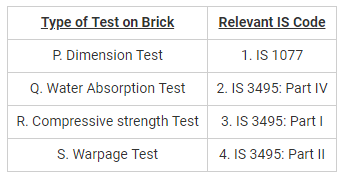Solution:

Dimension Test is done as per procedures laid down in IS 1077.

Water Absorption Test is done as per procedures laid down in IS 3495: Part II.

Compressive Strength Test is done as per procedures laid down in IS 3495: Part I.

Warpage Test is done as per procedures laid down in IS 3495: Part IV.

QUESTION: 6

The correct order of the following ingredients of Good Brick Earth with respect to its percentage composition in brick is:

Solution:

Composition of Good Brick earth are:

1. Silica = 50-60%

2. Alumina = 20-30%

3. Lime =  5 to 10%

4. Oxide of Iron = <5%

5. Magnesia = <1%

QUESTION: 7

The preservation of timber is done to:

Solution:

Preservation of timber is carried to achieve the following three objectives:

1. Increase the life of timber

2. Make the timber durable

3. Protect the timber structures from the attack of destroying agencies such as fungi insects.

QUESTION: 8

Deciduous trees are having

Solution:

Most deciduous trees have broad, well defined, wide and flat leaves. These leaves often change colour when they are about to shed. Deciduous trees are also tall and majestic in their form.These leaves fall in autumn and new ones appear in springs. They yield strong wood and hence they are commonly used in building construction.

QUESTION: 9

Which one of the following does not belong to exogenous tree?

Solution:

Exogenous trees are outward growing trees while Endogenous trees are inward growing trees. Some common examples are:

Endogenous Trees: bamboo, palm, cane etc.

Exogenous Trees: Pine, Fir, redwood, spruce, deodar, cedar, Maple, Mahogany, Oak, teak, walnut, babul etc.

Note: The wood derived from exogenous trees are mainly used for engineering purposes.

QUESTION: 10

The defect caused due to over-maturity and unventilated storage of the wood during its transit is called:

Solution:

Foxiness: This defect is due to the yellowish or reddish stains, caused by over maturity and lack of ventilation during storage.

Heart Shakes: Radial ruptures in annual rings or splits radiating from the heart and extending towards the sapwood are called heart shakes. Shrinkage of the interior part of the timber causes this crack.

Rind gall: Rind galls are curved swellings of trees which are formed at a point where a branch of the tress is improperly removed or fell down.

knot: Bases of branches or limbs which are broken or cut off from the tree are known as Knots.

QUESTION: 11

The measurement of Glazing is done in:

Solution:

The measurement of glazing is done in square meter. The various kinds of glass shall be described in accordance with usual terms and each shall be measured separately. Measurement of glazing in wood and metal is done separately.

QUESTION: 12

The rough cost estimate for a building having Plinth area and Plinth area rate as 700 square meter and 9000 Rs respectively is?

Add 15 % of the estimate as additional charges for public health and electric services also.

Solution:

Rough estimate of a building based on plinth area will be:

C1 = Plinth area x Plinth area rate

C1 = 700 x 9000 = 6300000 Rs.

Additional 15 % charges for public health and electric services,

C2 = 0.15 x 6300000 = 945000 Rs

Total rough estimate of the building = C+ C2

= 6300000 + 945000 = 7245000.

QUESTION: 13

Calculate the cost of the plastering required for a wall of 6 m long, 3.75 m high and 350 mm thick, if the rate of plastering is Rs. 17 per square meter.

Solution:

The cost of Plastering will be:

Area of the wall = 6 x 3.75 = 22.5 square meter

Rate of Plastering per square meter = Rs 17

Cost of Plastering the 22.5 square meter area of wall = Rs 382.5

QUESTION: 14

Which of the following area is included in the plinth area of the building?

Solution:

Plinth area is the covered built-up area measured at the floor level of any storey or at the floor level of the basement.

Following areas are included during measurement of plinth area:

1) Area of the wall at the floor level, excluding plinth offsets.

2) Areas of the internal shaft for sanitary installations and garbage chute, electrical, telecom and firefighting services

3) Vertical duct for air conditioning and lift well including landing

4) Staircase room or head room other than terrace level

5) Area of barsati at terrace level

Following areas are not included during measurement of plinth area:

1) Additional floor for seating in assembly buildings, theatres, auditoriums

2) Cantilevered porch, Balcony, Area of loft, Internal sanitary shaft and garbage shaft

3) Area of architectural band, cornice, Open platform etc.

4) Towers, turrets, domes projecting above the terrace level at terrace

QUESTION: 15

Find the book value of the machine at the end of 6 years if a machine is purchased for Rs. 15,00000 and has estimated life of 15 years.

Given salvage value (Rs) at the end of 15 years is 2,25,000. Use straight line method of evaluation of depreciation.

Solution:

Annual Depreciation is given by: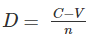C = Cost Price of Machine = Rs 15,00000

V = Salvage value = Rs 2,25,000

n = estimated life of machine in years = 15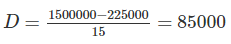Book value (B) is given by:

B = C - nD

n = No. of years after which Book value is to be computed

B = 1500000 - 6 x 85000

B = 990000

QUESTION: 16

Consider the following statements:

A. Stores and Balcony are included in the calculation of carpet area.

B. Staircases within the Property unit are included in carpet area

C. Roof Terrace are excluded from the carpet area.

The correct statements are:

Solution:

Carpet area is the usable area within the walls of the property unit where we can lay carpet.

Items Included in the carpet area of the building are:

i) Rooms: Living rooms, Bedrooms, study, dining room, Dressing room, Other room etc.

ii) Kitchen and Bathrooms

iii) Stores and Balconies

iv) staircases within the property unit

Items not Included in the carpet area of the building are:

i) External & Internal walls

ii) Utility ducts

iii) Common areas

iv) Terrace

QUESTION: 17

Accuracy in the measurement of the thickness of the slab or sectional dimension of column and beam (in centimetre) should be __________.

Solution:

Dimensions shall be measured nearest to a cm except for the thickness of slab, sectional dimension of column and beam which shall be measured correct to 0.5 cm. The areas shall be worked out nearest to 0.01 sq.mt. The cubical contents shall be worked out to nearest 0.01 cubic metre.

QUESTION: 18

Clam shell is a machine used for

Solution:

Clam shell is a machine used for Excavating. Clam shell is a bucket or grapple (as on a dredge) having two hinged jaws.

Hoe can be used to excavate relatively stiffer earth from a depth below the standing level of the equipment.

Drag line can be used to collect the loose material from a much larger depths.

Power shovel can be used to excavate the loose earth material effectively and can be used in undulating grounds because it has a crawler mounting.

QUESTION: 19

Most accurate method of estimation is based on:

Solution:

Detailed estimate is the most accurate method and consist of working out the quantities of each of item of works and working the cost.

The dimensions are taken directly from the drawings and quantities of each item are calculated.

Finally abstracting and billing is done.

QUESTION: 20

For 12 mm thick cement plastering 1:6 on 100 sq. m, new brick work, the quantity of cement required is _____.

Solution:

Plastering thickness 12 mmc= 12/1000 = 0.012m

Volume of cement mortar required = (Plastering Area x thickness) = 100 m2 x 0.012m = 1.2 m3

(This is wet volume that means we need this much volume of cement mortar after mixing water, So for dry volume, we have to add 30-35% as bulkage of sand, we are using 35% and wastage as 20%)

Consider 35% Sand Bulkage = 1.2 m3 x (1 + 0.2 + 0.35) = 1.86 m3

Cement required = (1/7) x 1.86 = 0.265 m3

QUESTION: 21

Which one of the following sets of internal angles (degree) of a triangle show well condition triangle?

Solution:

A triangle is stated to be a well-conditioned triangle when no angle in it is less than 30o or greater than 120o.

A triangle in which any angle of the triangle is less than 30o or greater than 120o is known as ILL conditioned triangle.

Option 4 alone fulfil the condition of well condition triangle.

QUESTION: 22

The staff reading at a distance of 80 m from a level with a bubble at centre is 1.31 m. When the bubble is moved 5 divisions out of the centre, the reading is 1.39 m. Then the angular value of one division of the bubble is:

Solution:
QUESTION: 23

If the Magnetic bearing of line is 52°40’ and magnetic declination is 4°20’ W, then the true bearing will be

Solution:
QUESTION: 24

Which of the following quantities are each equal to one acre?

P. 43560 sq ft

Q. 40 gunthas

R. 10 sq gunter’s chain

S. 4850 sq yds

Solution:

1 acre = 4850 sq yds

1 acre = 43560 sq ft

1 acre = 40 gunthas

1 gunter’s chain = 66 feet

1 square gunter’s chain = 66 × 66 = 4356 ft2

10 square gunter’s chain = 4356 × 10 = 43560 ft2

Also,

1 acre = 43560 sq ft

So, 1 acres = 10 square gunter’s chain

QUESTION: 25

In levelling between two points A and B on opposite banks of a river, the following reading were taken: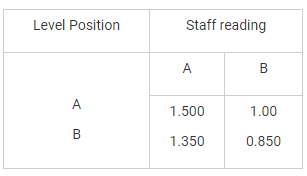If the R.L of A is 100.0 m, the R.L of B is

Solution:
QUESTION: 26

In a closed loop traverse of 1 km total length, the closing errors in departure and latitude are 0.3 m and 0.4 m respectively. The relative precision this traverse will be

Solution:

Total closing error(c)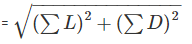Sum of latitude = 0.4 m

Sum of Departure = 0.3 m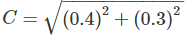C = 0.5m

Relative error of closure or Relative precision of this traverse  = c/p

p ⇒ Perimeter of traverse = 1 km = 1000 m

Relative precision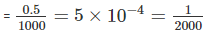QUESTION: 27

Which of the following surveys employs alidade?

Solution:

Instruments used in Plane Table surveying are:

2. Plumb bob and Plumb fork

3. Compass

4. Spirit level

5. Chain

6. Ranging rod

7. Tripod

Note: Alidade is a straight edge ruler having some form of sighting device. One edge of the ruler is bevelled and is graduated. Always this edge is used for drawing line of sight.

QUESTION: 28

Theory of error and adjustments deals with minimizing the effect of

Solution:

Systematic or cumulative errors which occur from well-understood causes can be reduced by adopting suitable methods. It follows some definite mathematical or physical law and a correction can be determined and applied.

Accidental errors are those which remain after mistakes and systematic errors have been eliminated and are caused by a combination of reasons beyond the ability of the observer to control.

Personal errors arise from the limitations of the human senses such as sight, touch and hearing.

Both accidental and personal error represents the limit of precision in the determination of a value. They obey the law of probability and therefore the theory of errors and adjustments applies to them.

QUESTION: 29

In a solution of the three-point problem in plane table surveying, the converging of error is attained through:

Solution:

In the three-point problem, if the orientation of the plane table is not proper, the intersection of the resectors through the three points will not meet at a point but will form a triangle, known as the triangle of error. The size of the triangle of error depends upon the amount of angular error in the orientation. This triangle of error will reduce to a point by trial and error.

QUESTION: 30

The following measurements were made during testing a levelling instrument.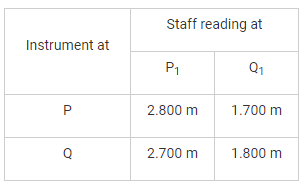P1 is close to P and Q1 is close to Q. If the reduced level of station P is 100.00 m, the reduced level of station Q is

Solution: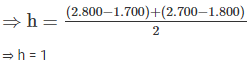∴ Reduced level of station,

Q = Reduced level of P + h

= 100.00 + 1 = 101.000 m

QUESTION: 31

The void ratio of a soil sample is given by 0.58. What is the porosity of soil sample?

Solution:

Porosity of soil sample is given by: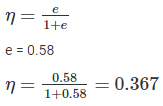QUESTION: 32

The maximum dry density and optimum moisture content of a soil is given by 1.65 gm/cc and 20.5% respectively. What is the percentage of air content of soil at OMC, if the specific gravity of particles is given by 2.65?

Solution:

Using the relation,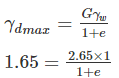e = 0.606

Using the relation

se = wG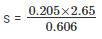s = 0.896

ac + s = 1

where, ac = air content

a= 0.104 = 10.4 %

QUESTION: 33

If seepage is taking place through a cohesionless soil the grains will be lifted if

Solution:

If flow is taking place in upward direction and the hydraulic gradient under this condition is greater than critical hydraullic gradient then the grains will be lifted i.e.

i > ic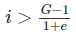QUESTION: 34

Toughness index of a soil is defined as the ratio of

Solution:

Toughness index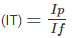Where Ip = Plasticity index

If – Flow index

QUESTION: 35

Identify the type of soil for a plasticity Index equal to 75.

Solution: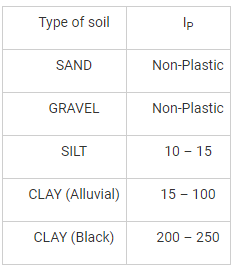QUESTION: 36

Double hemispherical buckets are used in

Solution:
QUESTION: 37

The flow at critical depth in an open channel is _____.

Solution:

For a given value of specific energy, the critical depth gives the greatest discharge in an open channel, or conversely, for a given discharge, the specific energy is a minimum for the critical depth. So at a control section, the discharge can be calculated once the depth is known e.g. The critical depth meter.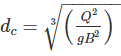Where B is the channel width.

QUESTION: 38

The specific speed of a turbine is the speed of such a turbine, identical with a given turbine, which

Solution:

Specific speed: It is defined as the speed of a similar turbine working under a head of 1 m to produce a power output of 1 kW. The specific speed is useful to compare the performance of various type of turbines. The specific speed differs for different type of turbines and is same for the model and actual turbine.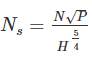QUESTION: 39

The stream function ψ = x3- y3 is observed for a two-dimensional flow field. What is the magnitude of the velocity at point (1, -1)?

Solution: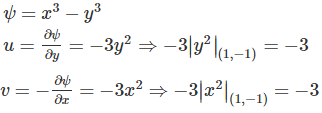Resultant velocity at (1,-1):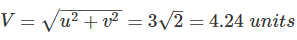QUESTION: 40

A mono pump is also knows as;

Solution:

Another form of positive acting rotary pump is the single-screw extruder pump typified by the Mono pump. It has a specially shaped metal helical rotor revolves eccentrically within a resilient rubber or plastic double helix, thus creating a continuous forming cavity which processes towards the discharge of the pump. This type of pump is suitable for pumping slurries and pastes, weather Newtonian or Non-Newtonian in character.

QUESTION: 41

Which one of the following statement is CORRECT about the centre of buoyancy?

Solution:

Objects in a fluid experience an upward force. Whenever an object is immersed in a fluid, either liquid or gas, it experiences a buoyant force. A buoyant force is a force which pushes upward on an object and is caused by displaced fluid.

The centre of buoyancy is the point where the resultant Buoyant force acts, It is the point of the C.G of the displaced fluid.

QUESTION: 42

The value of coefficient of velocity for a sharp-edged orifice is of the order of

Solution:
• Coefficient of velocity is defined as the ratio between actual velocity of a jet of liquid at vena-contracta and the theoretical velocity of jet.
• It varies from 0.95 to 0.99 for different orifice, depending on the shape, size of the orifice and on the head under which flow takes place.
• The value of coefficient of velocity is 0.98 for sharp edged orifice.
QUESTION: 43

Which of the following instruments is used to measure flow on the application of Bernoulli’s theorem:

Solution:

Flow rate through a pipe is usually measured by providing a coaxial area contraction within the pipe and by recording the pressure drop across the contraction, which is the application of Bernoulli’s equation.

Three flow meters primarily operate on this principle:

• Venturimeter
• Orificemeter
• Pitot tube
QUESTION: 44

In case of a strong hydraulic jump, the Froude Number is minimum of:

Solution:
QUESTION: 45

Irrigation canals are generally aligned along:

Solution:

The dividing ridgeline between the catchment areas of two streams (drains) is called the watershed or ridge canal. Thus between two major streams, there is the main watershed (ridge line), which divides the drainage area of the two streams. Similarly, between a mainstream and any of its tributary, there are subsidiary watersheds (ridge lines), dividing the drainage between the two streams on either side. The canal which is aligned along any natural watershed (ridge line) is called a watershed canal, or a ridge canal. Aligning a canal (main canal or branch canal or distributary) on the ridge ensures gravity irrigation on both sides of the canal. Since the drainage flows away from the ridge, no drainage can cross a canal aligned on the ridge. Thus, a canal aligned on the watershed saves the cost of construction of cross-drainage works.

QUESTION: 46

Consider the following statements:

1. In a super-passage, the drain runs over the canal.

2. In a siphon, the drain runs below the canal.

3. In a siphon aqueduct, type-II, the canal banks are made of RCC walls.

Which of these statements are correct?

Solution:

A super passage is just like a bridge in which the natural drain is carried over the canal. A super passage is constructed where the bed of the drain is well above the canal F.S.L.

A syphon serves the same purpose as super-passage and is constructed where the F.S.L. of the canal is higher than the bed of the drain.

Aqueducts are of the following three types:

Type-I: In this type, the sides of the aqueduct (or syphon aqueduct) are earthen banks with complete earthen slopes.

Type-II: In this type also the canal continues in its earthen section over the drain, but the outer slopes of the earthen banks are replaced by retaining walls.

Type-III: In this type, the earthen banks are not provided through the aqueduct (or syphon aqueduct) and the canal water is carried in a masonry or concrete trough.

QUESTION: 47

Calculate the safe stopping sight distance for a design speed of 60 km/h for two-way traffic on a single lane road. The reaction time of driver is 2.5 sec.

Solution:

For one-way traffic, SSD is given by: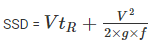Where,

f = coefficient of longitudinal friction = 0.35

g = acceleration due to gravity

V = Design speed = 60 Kmph = 0.278 × 60 m/s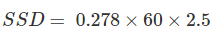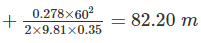Now for two-way traffic,

SSD required = 2 × SSD = 2 × 82.20 = 164.40 m

QUESTION: 48

Seal coat in a bituminous road is

Solution:

Seal coat in a bituminous road is a mixture of coarse sand and bitumen. Seal coat serves to seal the top of the asphalt, preventing water from penetrating the surface of the pavement and protecting the top layer of asphalt from oxidation and wear caused by exposure to the sun and air. Seal coat also beautifies the pavement by providing a smooth, black, even surface ideal for painting lines and sweeping.

QUESTION: 49

Given that the width of the sleepers = w, the sleeper spacing = s, then the depth of ballets ‘d’ is

Solution:
QUESTION: 50

The trap which is provided to disconnect the house drain from the street sewer is called ________.

Solution:

The master trap or intercepting trap is provided to disconnect the house drain from the municipal sewer and to prevent the foul gases from municipal sewer.

QUESTION: 51

Which one of the following is not a coagulating agent?

Solution:

Copper sulphate is not a coagulating agent. Trivalent aqua metallic cationic chemical substances have high coagulating power. The coagulants are –

1. Aluminium sulphate (Alum)
2. Ferrous sulphate (Copperas)
3. Ferric sulphate & Ferric chloride (Chlorinated copperas)
4. Sodium aluminate
QUESTION: 52

Pick up the incorrect statement from the following.

Solution:

Inlets are generally provided in all sewers.

Manholes are provided in sewer pipes where the diameter of sewer changes or the direction of flow changes.

Catch basins are generally provided in the sewer for segregation of solid particles from drained water.

QUESTION: 53

Generally, water is satisfactorily disinfected, if the free available residual chlorine is about __________mg/litre at the end of 10 minutes contact periods.

Solution:

Generally water is satisfactory disinfected if the free available residual chlorine is about 0.2 mg/lit. When free Cl2 residual excess present in water and comes in contact with organic substances produce THM (Tri-halo-methane) in water which are carcinogenic in nature. Therefore free chlorine residual  if present above 0.2 mg/lit there is a risk of THM formation and if present less than 0.1 mg/lit, no future protection.

QUESTION: 54

On which of the following parameters are the slow sand filters better than rapid sand filters?

Solution: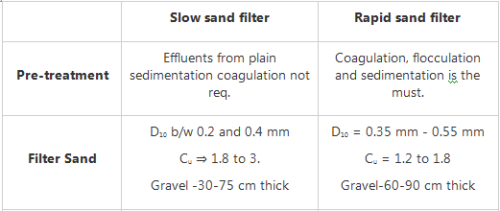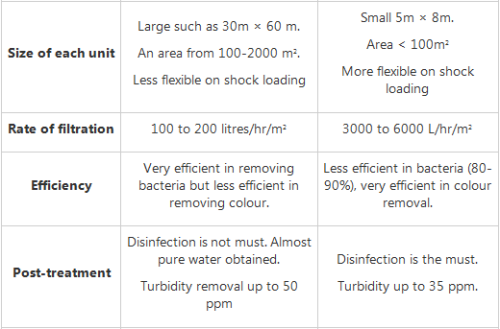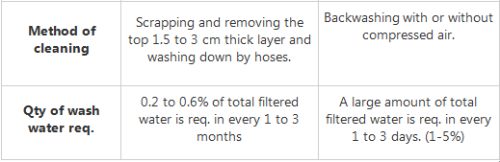QUESTION: 55

To connect members subjected to dynamic loads which one of the following type of bolts is preferable?

Solution:

In bearing type of connections, the plates are in firm contact but may slip under loading until the hole surface bears against the bolt. Under dynamic loads, the nuts are liable to become loose and so black bolts are not allowed for use under such loading. In situations where small slips can cause significant effects as in beam splices, black bolts are not preferred. High Strength Friction Grip bolts (HSFG) provide extremely efficient connections and perform well under fluctuating/fatigue load conditions.

QUESTION: 56

In the T-section, as shown in the figure (all dimensions are in mm), the distance of plastic neutral axis from the top is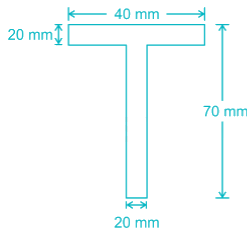Solution:
QUESTION: 57

Two steel plates, at right angle are welded with fillet weld of 16 mm size. The throat thickness of fillet weld should be

Solution:

Throat thickness= 0.7 × size of weld

= 0.7 × 16 = 11.2 mm.

QUESTION: 58

For a Plate Girder, diagonal buckling of the web occurs when the ratio of clear depth to the thickness of web exceeds

Solution:

For a Plate Girder, diagonal buckling of the web occurs when the ratio of clear depth to the thickness of the web exceeds 85.

So when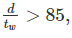stiffeners are provided.

QUESTION: 59

If the yield stress of a structural member made of steel is fy = 230 N/mm2. Then which of the following are correctly matched.

Solution:

Permissible tensile stress in axial tension = 0.6 fy = 138 N/mm2

Permissible bending stress in tension = O.66 fy = 151.8 N/mm2

Permissible shear stress = 0.4 fy = 92 N/mm2

QUESTION: 60

Which of the following is NOT a method recommended by IS 800 for assessing the period of structural adequacy of structural steel design?

Solution:

As per IS 800: 2007, clause 16.3.2, the period of structural adequacy of structural steel design can be assessed by the following methods:

1. By calculating the limiting temperature of the steel
2. By direct application of the single test
3. The methods given in the specialist literature
QUESTION: 61

A member subjected to compressive loads resulting from wind/earthquake forces provided the deformation of such members does not adversely affect the stress in any part of the structure. The maximum slenderness ratio is

Solution: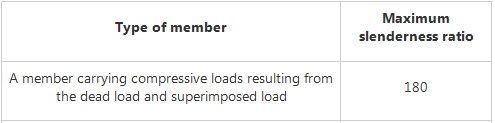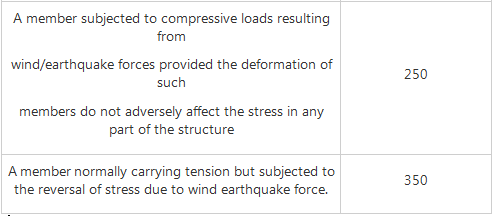QUESTION: 62

The concrete sample is cured at 15 degree Celsius for 28 days. If the origin temperature is taken as -11 degree Celsius, what is the maturity (degree Celsius days) of concrete sample?

Solution:

Correct Expression for maturity of the concrete is:

M = ∑ (Time × Temperature)

-11°C is taken as a datum line for computing maturity.

M = 15 x 28 + 11 x 28 = 7280C days.

QUESTION: 63

On which of the following does the correct proportion of ingredients of concrete depend upon?

Solution:

When bulking is not accounted for proportioning a mix by volume, then use of moist sand will result in less quantity of sand than calculated. This decrease in batch quantity of sand will reduce the yield of concrete per bag of cement. That's why Bulking is taken in to consideration in proportioning of concrete.

QUESTION: 64

In which of the following cases, steam curing should be preferably be used?

Solution:

Steam curing substantially reduces the time for attaining strength for any concrete. For pre-casting, the time for concrete to attain strength is considered as idling time. If this time is minimized, we can have more precast elements produced in less time using the same set of moulds. Hence steam curing is always helpful for pre-casting.

QUESTION: 65

For given water content, workability decreases if the concrete aggregates contain an excess of

Solution:

All of the above options are correct.

Thin particles, flat particles and elongated particles has more surface area, therefore more water is needed to get the workable paste of concrete. So for given water content, workability decreases for the above case.

QUESTION: 66

Which of the following is not a direct advantage of using concrete pumps for pumping concrete?

Solution:

Concrete pumping is the most accurate and quality way of pouring concrete exactly where it is needed.

Benefits of using Concrete pumps are:

1. Increase Concrete Pouring Speed

2. Reduce the Number of Labours Needed

3. Ensure higher accuracy and quality in concrete pouring

4. Overall increase in productivity of construction.

Note: As there is a slight increase (negligible) in concrete strength is observed because Concrete pumps required less amount of water compared to other concrete pouring techniques, thus decrease in water cement ratio will lead to increase in strength of concrete.

From all the above options, most appropriate answer is option 3.

QUESTION: 67

The bottom diameter and top diameter of the steel mould used for slump test are respectively:

Solution:
QUESTION: 68

The addition of surfactants in the concrete mix results in:

i). Increase in the water cement ratio

ii). Decrease in the water cement ratio

iii). Increase in the strength of concrete

iv). Decrease in the curing duration

v). Increase in the density of concrete

Which one of the following statements is correct?

Solution:

The introduction of suitable surfactants into the concrete mix results in the formation of fine and stable air bubbles. When the fresh mixture begins to cure, the bubbles within it become mineralized and become an integral part of it. In this way, an additional space is created inside the concrete structure inside which the freezing water expands.

This prevents an increase in internal pressure, which is responsible for the formation of cracks during low temperatures.

As the voids in concrete are reduced, so less water is required to lubricate the mix, thus water cement ratio will be decreased, and strength of concrete will be increased. Addition of surfactants will increase the density of concrete.

QUESTION: 69

The difference in shrinkage between surface and interior of concrete results in:

Solution:

Crazing: Crazing in concrete is the development of a network of fine random cracks or fissures on the surface of concrete caused by shrinkage of the surface layer. These cracks are rarely more than 3mm deep. They do not affect the structural integrity of concrete.

Poor or inadequate curing, Excessive laitance on surface and Sprinkling cement on the surface to dry up the bleed water are the common causes of crazing in concrete.

Efflorescence: Efflorescence is a crystalline deposit of salts that can form when salty water is present in or on brick, concrete, stone, stucco or other building surfaces. It has a white or greyish tint and consists of salt deposits left behind when water evaporates.

Bleeding: Bleeding is one form of segregation, where water comes out to the surface of the concrete, being lowest specific gravity among all the ingredients of concrete. It seriously affects the durability and strength of concrete.

Segregation: Segregation of concrete is separation of ingredients of concrete from each other. In case of segregation, the heavy aggregate particles settle down leaving a sand cement mix on top affecting the quality adversely.

QUESTION: 70

Which of the following is not natural puzzolana?

Solution:

Surkhi is broken brick powder or burnt clay soil and used as a substitute for sand for concrete and mortar, and has almost the same function as of sand but it also imparts some strength and hydraulicity.

QUESTION: 71

To obtain very high strength concrete, it is necessary to use very fine grained:

Solution:

Coarse aggregates are obtained by crushing various types of granites (such as syenites, dolerites, quartzites etc), when very high strength concrete is required, a very fined grained granite is perhaps the best aggregate.

QUESTION: 72

The quantity of cement required in 1 cubic meter of 1:2:4 cement concrete is approximately:

Solution:

Volume of concrete = 1 cu m

Since 1:2:4 represents 1 part of cement, 1 part of sand, 1 part of aggregates in cement concrete

Dry volume of concrete = 1.54 x wet volume of concrete

Wet volume of concrete = 1 cu m

Vdry = 1.54 cu m

Volume of cement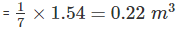QUESTION: 73

Creep deformation is a property of any material to deform under the influence of:

Solution:

Creep is the tendency of a solid material to move slowly or deform permanently under the influence of mechanical stresses. Creep may also be known as material creep or cold flow. It is basically a time- dependent deformation under a certain applied load.

Stresses due to wind loads are varying with time and we know creep is due to sustained loads, therefore no creep deformation is observed due to wind loads.

Thermal stresses are also acting over the short time, so no creep deformation is observed due to wind loads also.

QUESTION: 74

According to IS 382: 1963, a good aggregate should be:

Solution:

For a good concrete mix, aggregates need to be clean, hard, strong particles free of absorbed chemicals or coatings of clay and other fine materials that could cause the deterioration of concrete.

According to IS 382: 1963, a good aggregate should be chemically inert, sufficiently strong, hard and durable etc.

QUESTION: 75

Which of the following Indian standard is referred to determine zone of fine aggregate:

Solution:

IS 383: Coarse and Fine Aggregate for Concrete – Specification

Fineness modulus limits for various zones of sand is given in IS 383. Example-For 4.75 mm IS sieve, if fineness modulus is in between 90-100, then that sand lies in zone 1.

IS 456: Plain and Reinforced concrete code of practice.

IS 1237: Cement Concrete Flooring Tiles – Specification.

IS 1893: Criteria for Earthquake Resistant Design of Structures

QUESTION: 76

The bulk density of aggregates does not depend upon:

Solution:

Bulk density is the important parameter to obtain the useful information regarding the shape and grading of aggregates.

Size and Shape of aggregate: Bulk density of aggregate is inversely proportional to size of aggregate. Bulk density of aggregate depends upon shape (rounded, elongated etc) of aggregate, with increase in surface area of aggregate bulk density of aggregate decreases. For coarse aggregate, higher bulk density indicates a fewer voids to be filled by cement and sand.

Higher grading of aggregate will lead to decrease in voids of aggregate which in turn increases the bulk density of aggregate.

Specific gravity: Specific gravity of aggregate is directly proportional the bulk density of aggregate.

Note: Bulk density of aggregate does not depend upon the size and shape of the container.

QUESTION: 77

Which of the following constituents is present in the blast furnace slag?

Solution:

Constituents present in blast furnance slag are: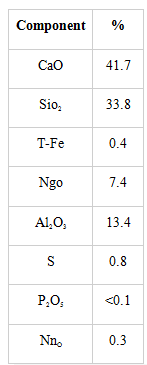QUESTION: 78

Single Flemish bond consist of

Solution:

This bond is a combination of English bond and Flemish bond. In this work the facing of the wall consists of Flemish bond and the backing consists of English bond in each course. This type of bonding cannot be adopted in walls less than one and a half brick in thickness. This bond is adopted to present the attractive appearance of Flemish bond with an effort to ensure full strength in the brick work.

QUESTION: 79

Which of the below structure doesn’t require Pre Stressed Concrete?

Solution:

Prestressing is done to reduce or eliminate the internal tensile stresses by applying an initial compressive axial force to the concrete. It does this by tensioning the steel cable running through the concrete. The steel cable is then anchored and a compressive force is transferred to the concrete by bond forces.

But in case of arch, tensile stresses do not occur in the structure, all compressive forces evolve directly from the load due to its specially designed shape. The arch form is one of the earliest solutions to dealing with a material which has no tensile strength.

QUESTION: 80

Long and short spans of a two-way slab are ly and lx and load on the slab acting on strips parallel to lx and ly be wx and wy respectively. According to Rankine Grashoff theory

Solution:
QUESTION: 81

The estimated flexural strength (MPa) of a concrete having a measured compressive strength of 64 MPa would be (as per IS 456)

Solution:

As per IS 456 : 2000, the estimated flexural strength (fct) of concrete is given by: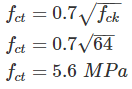QUESTION: 82

Consider the following statement regarding characteristic strength of concrete:

“The test result of the sample shall be the average of the strength of x specimens. The individual variation should not be more than Y% of the average,”

What shall be the values of X and Y?

Solution:

As per IS 456 : 2000, Clause 15.4:

The test results of the sample shall be the average of the strength of three specimen. The individual variation shall not be more than 15% of the average, If more test results of the sample will be considered as invalid.

QUESTION: 83

The modulus of elasticity of steel is more than that of concrete. It indicates that steel is

Solution:

The Modulus of elasticity has no relation with plasticity of the material, Therefore, no conclusion can be drawn with reference to plasticity of the material for both. As the modulus of elasticity of steel is more than that of concrete, so steel is more elastic than concrete.

QUESTION: 84

R.C.C. was developed and first used by:

Solution:

Francois Coignet was a French industrialist of the nineteenth century. He was a pioneer in the development of structural prefabricated and reinforced concrete. Coignet was the first to use iron-reinforced concrete as a technique for constructing building structures. In 1853 he built the first iron reinforced concrete structure anywhere, a four-story house in paris.

Joseph Monier was a French gardener and one of the principal inventors of reinforced concrete. Joseph Monier patented a method of strengthening thin concrete flowerpots by embedding iron wire mesh into the concrete.

John Smeaton was a British civil engineer responsible for the design of bridges, canals, harbours and lighthouses.

Joseph Aspdin was an English cement manufacturer who obtained the patent for Portland cement on 21 October 1824.

QUESTION: 85

Enlarged head of a supporting column of a flat slab is technically known as _____.

Solution:
QUESTION: 86

A short column 20 cm x 20 cm in section is reinforced with 4 bars whose area of cross section is 20 sq.cm. If permissible compressive stresses in concrete and steel are 40 kg/cm2 and 300 kg/cm2, the safe load on the column should not exceed ________.

Solution:

The load carrying capacity will be,

W = Stress in concrete x area of concrete + stress in steel x area of steel

W = 40 x (20 x 20 - 20) + 300 x (20)

= 21200 Kg

So none of these are correct.

QUESTION: 87

The shear force diagram (SFD) for the beam shown in figure is: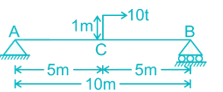Solution:
QUESTION: 88

In the “T” section as shown in figure, distance of neutral axis from the top is: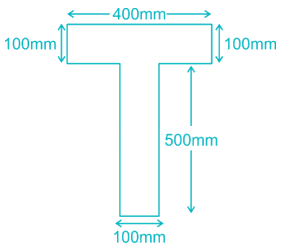Solution:
QUESTION: 89

A right circular cone of base diameter 3 m and height 4 m having weight of 2 kN. What is the distance of its centre of gravity above the base?

Solution:
QUESTION: 90

A simply supported beam of span ‘L’ and uniform flexural rigidity EI carries a central load ‘W’ and total uniform distributed load ‘W’ throughout the span. The maximum deflection is given by.

Solution:
QUESTION: 91

Find the tensile stress of a mild steel rod of 18 mm diameter. Given: ultimate load = 9.0 ton.

Solution:

Tensile stress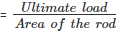Area of the rod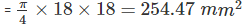Tensile stress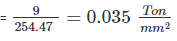QUESTION: 92

The ratio of the slenderness ratio of a vertical column of square cross-section of side 15cm to the slenderness ratio of a vertical column of circular cross-section of diameter 15cm having Same effective length will be?

Solution:
QUESTION: 93

The temperature stress is a function of

1. Coefficient of linear expansion

2. Temperature rise

3. Modulus of elasticit

Solution:

Thermal strain ϵis proportional to the temperature change ΔT

ϵT = αΔT

α is coefficient of thermal expansion.

When there is some restriction to the bar to expand, thermal stress will generate in the material:

σT = strain×E = α.ΔT.E

QUESTION: 94

A state of pure shear in a biaxial stress in given by

Solution:
QUESTION: 95

The material in which large deformation is possible before the absolute failure or rupture is termed as

Solution:

The material in which large deformation is possible before the absolute failure or rupture is termed as ductile. Ductility is the characteristic which permits a material to be drawn out longitudinally to a reduced section, under the action of tensile force.

QUESTION: 96

Assume that young's modulus of a wire of length L and radius 'r' is Y. If length is reduced to L/2 and radius r/2 then what will be its young's modulus?

Solution:

Young's is a property of the material of construction of a wire. It depends only on the nature of the material used for making the wire.

Young's modulus does not depend on the physical dimensions such as the length and diameter of wire. Thus there will be no change in Young's modulus.

QUESTION: 97

In a thin cylinder subjected to internal pressure, if lognitudinal stress is 30 N/mm2, circumferential stress is _________

Solution:

In thin cylinder longitudinal stress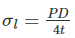Circumferential / hoop stress,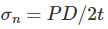∴ Hoop stress σn = 2σl

= 2 × 30

= 60 N/mm2

QUESTION: 98

A symmetrical parabolic arch of span 20 metres and rise 5 m is hinged at its springings and crowns and is subjected to uniformly distributed load of 100 kN/m throughout the span. The horizontal thrust at springings will be

Solution:
QUESTION: 99

The magnitude of fixed end moment in a fixed beam of span ‘l’ at A subjected to a loading as shown in figure below is?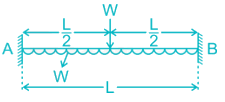Solution:
QUESTION: 100

For a Plane frame shown in the figure below, the Degree of Kinematic indeterminacy will be?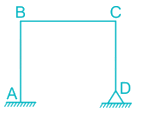Consider Member BC to be inextensible.

Solution:

Degree of Kinematic indeterminacy is given by:

Dk = 3J – re – m

J → no. of Joints

re → Reactions present at that Joint

m → no. of inextensible members

J = 4

re = 5

m = 1

Dk = 3 × 4 – 5 – 1

Dk = 12 – 5 – 1

Dk = 6